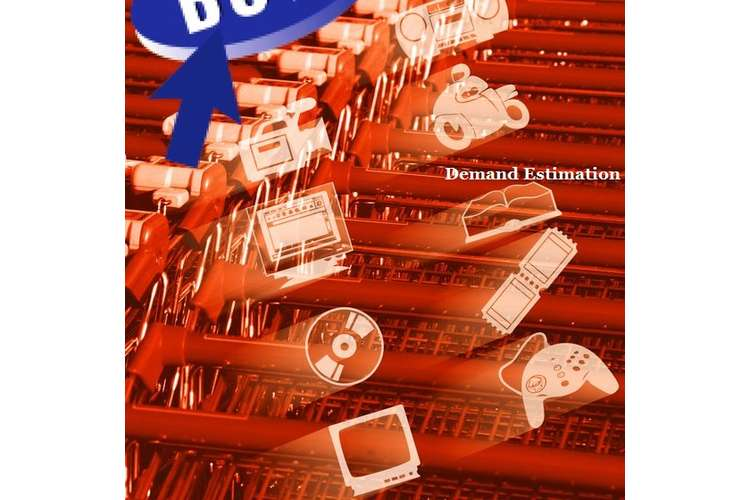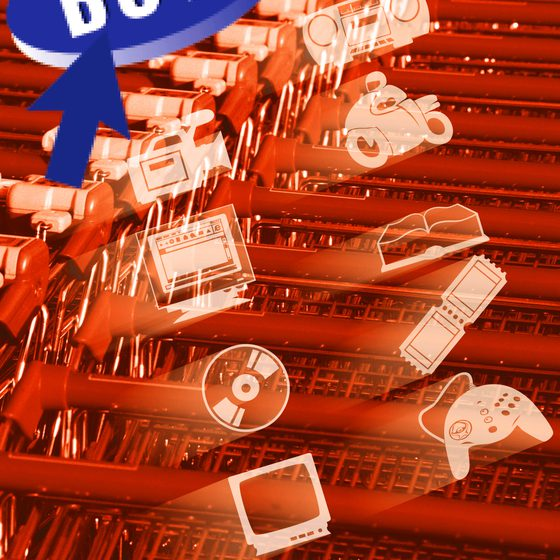# ECO550 Demand Estimation Editing Services### ECO550 Demand Estimation Editing Services

This ECO550 demand estimation assignment solution discuss emphirical demand function determine the dependent and independent variables and managerial economics.

## Introduction

Jiffy Med is a firm that produces and sells several medical products, one of the main products being Quick Kit. The product has one serious competitor, i.e. Headset manufactured by BigPharma. The current price of Quick Kit is \$30.00 and the marginal costs of production is nil for the product. Hence, it is important to understand the impacts of different factors on the overall demand for the product. Since the product is being marketed to the consumers, determining the benefits of the advertising expenditures of the firm is also a good option. The study further would elaborate on the impacts of different factors on the overall demand of the product, Quick Kit.### Estimation of the Empirical Demand Function

The first step in understanding the impacts of each of the factors is to determine the overall demand function for the product. The empirical demand function is nothing but a demand function that is made from the real market data. In order to determine and estimate the empirical demand function, the first thing that is important is to determine the dependent and independent variables. (Oum, et. al 1992) The dependent variable here is the quantity demanded of Quick Kit and the independent variables are the price of the product (P), average after-tax income of households(M), the overall population of the country(N), advertising expenditures(A) and the price of the competition product, Hepatest (P_h)

Hence, the empirical demand function can be demonstrated by the following equation:

Q = a + bP + cM + dN + eA + fP_h

We assume that the relationship is log linear because taking the log linear function helps in removing the elasticities from the demand function. (Ericsson, 1998) Hence, we take the log linear function of the same:

In Q = ln a + b ln P + c ln M+ d ln N + e ln A + f ln P_h

In order to determine the relationships, regression analysis is carried out, the data and results of which are given in appendices A and B respectively. From the results obtained from the regression analysis, the empirical demand function can be given as follows:

Q = 54172.2 – 466.8P + 0.137M -0.001N + 0.519A + 439.56 P_h

ln Q = -1.288 ln P + 0.316 ln M + 0.805 N + 0.1206 ln A + 0.44 P_h

### Interpretation of the Estimated Demand Function

The empirical demand function that has been estimated here gives a complete overview of the impacts of different factors as observed on the quantity demanded of QuickKit. The normal and the log linear demand functions, both demonstrate that there exists an inverse relationship between the quantity demanded and the price of the product. As the price increases, the demand would decrease and with a reduction in the price, the demand would increase. Another inverse relation is shown between the demand and the population, whereas the function demonstrates an increase in the demand with an increase in the household income, an increase in the advertising expenses and an increase in the competitive product.

Whereas the log linear function removes all elasticities of the demand curve and shows that the quantity demanded is positively correlated to average household income, Canadian population, advertising expense and the price of Hepatest, whereas the price exhibits a negative impact. This makes logical sense, as price goes up people would buy less but if the price of the competitive product goes up, the demand would automatically go down. Similarly, the quantity increases with population and when the household income increases. However, the extent of impact caused by each factor is demonstrated by the coefficients of each variable.

### Recommendations & Conclusion

Based on the estimated demand functions, it is recommended that the management should spend more on advertising. Advertising expenditures are shown to increase the overall demand for the product. Hence, it is obvious that spending on advertising is a smart move, as it leads to an increment in the demand. Though the impact has not been consistent throughout but the analysis of so many data points gives an estimate of the correlation. And hence Med should consider spending more on advertising because it would boost up demand even if other factors go down.

Also, with respect to the price it is obvious that the demand increases with decreasing prices and the extent of impact of the price on demand is quite high. Hence, in case the company wants to increase its sales and demand, it needs to bring down its prices. The data clearly indicates that places where prices have been high, the demand has been low and vice versa. Also, the marginal cost of producing each item is almost zero and the fixed costs would remain the same irrespective of the quantity produced. And hence, the company can reduce its prices because that would lead to higher demand and in situations where the marginal cost is nil, production and selling of more number of products would be beneficial.

Thus, it can be concluded that the demand function of Quick Kit does not only depends on the factors related to the firm and the product but also depends on the price of the other competitive product. And hence it is important for the senior manager to consider and keep this demand function in mind while making decision to ensure maximum benefits to the firm. Reducing prices and spending more on advertising can really help in increasing profits and benefits.

### References

1. Ericsson, N.R.(1998), Empirical Modeling of Money Demand, Empirical Economics, vol. 23, pp.295-315
2. Oum, T.H., Waters, W.G., & Yong, J.S.(1992), Concepts of Price Elasticities of Transport Demand and Recent Empirical Estimates, Journal of Transport Economics and Policy, vol.26, no,2

OZ Assignment Help is leading assignment writing serviceprovider in australia. Get best assignment help form OZ expert team on all type of subject and project assignment help.